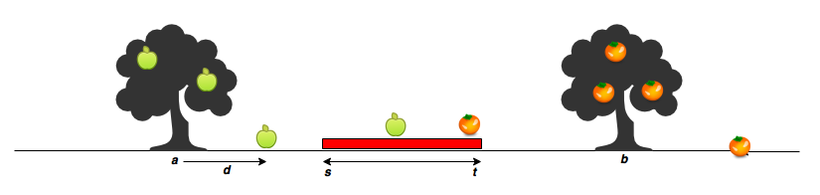# C Programming – HackerRank Solution | Apple and Orange Count

### Write a Modular C Programming code to find out the count of apple and orange fruits, which will fall on the Roof of Ram’s house.

Ram’s house has an apple tree and an orange tree. In the diagram below, the red region denotes his house, where s is the start point, and t is the endpoint. The apple tree is to the left of his house, and the orange tree is to its right. You can assume the trees are located on a single point, where the apple tree is at point a, and the orange tree is at point b.When a fruit falls from its tree, it lands d units of distance from its tree of origin along the x-axis. A negative value of d means the fruit fell d units to the tree’s left, and a positive value of d means it falls d units to the tree’s right. Given the value of d for 3 apples and 3 oranges, determine how many apples and oranges will fall on Ram’s house (i.e., in the inclusive range [s,t]?

ANALYSIS:

1. In the given problem we need to find out the count of apple and orange fruits, which will fall on the roof of Ram’s house.
2. Variables ‘s’ & ‘t’ represents the roof width of the house.
3. Get the distance ‘d’ of fruit from the corresponding tree.
4. Check whether ‘d’ is in between ‘s’ & ‘t’ so that fruit falls on the roof and increment the counter.

We must repeat steps 3 and 4 for all distances ‘d’ of fruits.

So we need to do a repetition of operations as mentioned in steps 3 and 4.Input Format

s t – width of Ram’s house roof (range)

a b – points on the x-axis where the apple and orange trees are there in the house yard.

m n – number of apples and oranges fall from respective trees.

d d d . . . – a distance of each apple filling.

d d d . . . – a distance of each orange falling.

Constraints

1 ≤ s,t, a, b, m, n ≤ 100000

-100000 ≤ d ≤ 100000

a < s < t < b

A negative value of d means the fruit falls d units to the tree’s left, and a positive value of d means it falls d units to the tree’s right.

Output Format

Counts of apples and oranges fall on Ram’s house.

Sample Input 0

```7 11
5 15
3 2
-2 2 1
5 -6
```

Sample Output 0

```Apple count = 1
Orange count = 1
```

Sample Input 1

```7 11
8 15
```

Sample Output 1

```Invalid input
```

Sample Input 2

```15 20
3 30
5 5
10 11 12 13 14
-10 -11 -12 -13 -14
```

Sample Output 2

```Apple count = 3
Orange count = 5```

CODE:

```#include<stdio.h>
#include<stdlib.h>
#include<string.h>
#include<math.h>

int main() {
int i,s,t,a,b,x,y,z,w,m,n,d1,d2,count=0,count1=0;
scanf("%d%d",&s,&t);
scanf("%d%d",&a,&b);
scanf("%d%d",&m,&n);
if(s>a&&t<b&&t>s)
{
for(i=0;i<m;i++)
{
scanf("%d",&d1[i]);
}
for(i=0;i<n;i++)
{
scanf("%d",&d2[i]);
}

for(i=0;i<m;i++)
{
if(d1[i]>0)
{
x=s-a;
w=t-a;
if(d1[i]>=x&&d1[i]<=w)

{
count++;
}
}
}
for(i=0;i<n;i++)
{
if(d2[i]<0)
{
y=t-b;
z=s-b;
if(d2[i]<=y&&d2[i]>=z)
{
count1++;
}
}
}

printf("Apple count = %d\n",count);
printf("Orange count = %d",count1);
}
else
printf("Invalid input");

}
```

OUTPUT

```Congratulations, you passed the sample test case.

Click the Submit Code button to run your code against all the test cases.

Input (stdin)

7 11
5 15
3 2
-2 2 1
5 -6

Apple count = 1
Orange count = 1
Expected Output

Apple count = 1
Orange count = 1```

Please find some more codes of Loops, Condition Statements, 1D Arrays, 2D Arrays, Strings, Pointers, Data Structures, Files, Linked lists, MISC, Solved model question papers & Hacker Rank all solutions on the below page:

Top 100+ C Programming codes – KLE Technological University